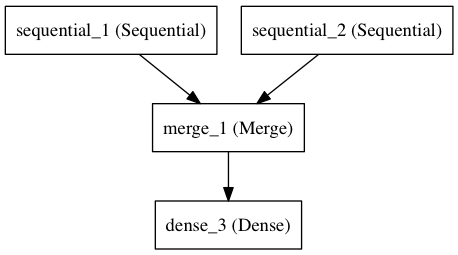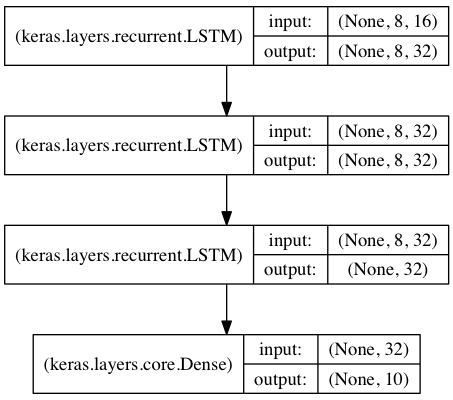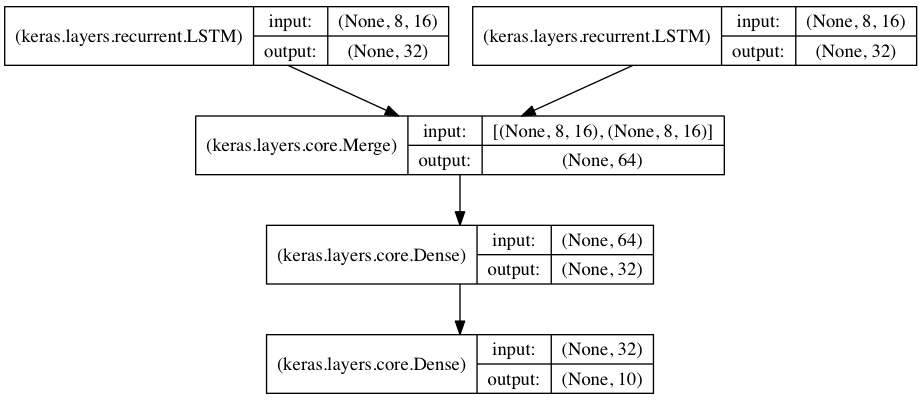2019/04/10 10:10

# Keras实现RNN模型

## SimpleRNN层

keras.layers.GRU(units, activation='tanh', recurrent_activation='hard_sigmoid', use_bias=True,
kernel_initializer='glorot_uniform', recurrent_initializer='orthogonal', bias_initializer='zeros',
kernel_regularizer=None, recurrent_regularizer=None, bias_regularizer=None, activity_regularizer=None,
kernel_constraint=None, recurrent_constraint=None, bias_constraint=None, dropout=0.0,
recurrent_dropout=0.0, implementation=1, return_sequences=False, return_state=False, go_backwards=False,
stateful=False, unroll=False)

• units：输出维度
• activation：激活函数（参考激活函数
• use_bias: 布尔值，是否使用偏置项
• kernel_initializer：kernel权重矩阵的初始化器，用于输入的线性转换（参见初始化器
• recurrent_initializer：recurrent_kernel 权重矩阵的初始化程序，用于循环状态的线性转换（参见初始化程序
• bias_initializer：偏置向量的初始化器（参见初始化器
• kernel_regularizer：应用于kernel权重矩阵的正则化函数（参见正则化器
• bias_regularizer：应用于偏置向量的正则化函数（参见正则化器
• recurrent_regularizer：应用于recurrent_kernel权重矩阵的正则化函数（参见正则化器
• activity_regularizer：应用于图层输出的正则化函数（其“激活”）。（见规范者
• kernel_constraints：应用于kernel权重矩阵的约束函数（请参阅约束
• recurrent_constraints：应用于recurrent_kernel权重矩阵的约束函数（请参阅约束
• bias_constraints：应用于偏置向量的约束函数（请参阅约束
• dropout：0~1之间的浮点数，控制输入线性变换的神经元断开比例
• recurrent_dropout：0~1之间的浮点数，控制循环状态的线性变换的神经元断开比例
• 其他参数参考Recurrent的说明

## GRU层

keras.layers.recurrent.GRU(units, activation='tanh', recurrent_activation='hard_sigmoid', use_bias=True,
kernel_initializer='glorot_uniform', recurrent_initializer='orthogonal',
bias_initializer='zeros', kernel_regularizer=None, recurrent_regularizer=None,
bias_regularizer=None, activity_regularizer=None, kernel_constraint=None,
recurrent_constraint=None, bias_constraint=None, dropout=0.0, recurrent_dropout=0.0)

• units：正整数，输出空间的维数
• 激活：要使用的激活功能（请参阅激活
• recurrent_activation：用于循环步骤的激活功能（参见激活）。
• use_bias：Boolean，该层是否使用偏向量
• kernel_initializerkernel权重矩阵的初始化器，用于输入的线性转换（参见初始化器
• recurrent_initializerrecurrent_kernel 权重矩阵的初始化程序，用于循环状态的线性转换（参见初始化程序
• bias_initializer：偏置向量的初始化器（参见初始化器
• kernel_regularizer：应用于kernel权重矩阵的正则化函数（参见正则化器
• recurrent_regularizer：应用于recurrent_kernel权重矩阵的正则化函数（参见正则化器
• bias_regularizer：应用于偏置向量的正则化函数（参见正则化器
• activity_regularizer：应用于图层输出的正则化函数（其“激活”）。（见规范者
• kernel_constraint：应用于kernel权重矩阵的约束函数（请参阅约束
• recurrent_constraint：应用于recurrent_kernel权重矩阵的约束函数（请参阅约束
• bias_constraint：应用于偏置向量的约束函数（请参阅约束
• dropout：浮点数介于0和1之间。为输入的线性变换而下降的单位的分数
• recurrent_dropout：浮点数在0和1之间。对于循环状态的线性变换，单位的分数下降
• implementation：实现模式，1或2.模式1将其操作构造为更大数量的较小点产品和添加，而模式2将其分组为更少，更大的操作。这些模式将在不同硬件和不同应用程序上具有不同的性能配置文件。
• return_sequences：布尔值。是返回输出序列中的最后一个输出，还是返回完整序列。
• return_state：布尔值。是否返回除输出之外的最后一个状态。
• go_backwards：Boolean（默认为False）。如果为True，则向后处理输入序列并返回相反的序列。
• stateful：Boolean（默认为False）。如果为True，则批次中索引i处的每个样本的最后状态将用作后续批次中索引i的样本的初始状态。
• unroll：Boolean（默认为False）。如果为True，则将展开网络，否则将使用符号循环。展开可以加速RNN，尽管它往往会占用大量内存。展开仅适用于短序列。
• reset_after：GRU约定（是否在矩阵乘法之前或之前应用复位门）。False =“之前”（默认），True =“之后”（CuDNN兼容）。

## LSTM

keras.layers.LSTM(units, activation='tanh', recurrent_activation='hard_sigmoid', use_bias=True,
kernel_initializer='glorot_uniform', recurrent_initializer='orthogonal', bias_initializer='zeros',
unit_forget_bias=True, kernel_regularizer=None, recurrent_regularizer=None, bias_regularizer=None,
activity_regularizer=None, kernel_constraint=None, recurrent_constraint=None, bias_constraint=None,
dropout=0.0, recurrent_dropout=0.0, implementation=1, return_sequences=False, return_state=False,
go_backwards=False, stateful=False, unroll=False)

• units：正整数，输出空间的维数
• 激活：要使用的激活功能（请参阅激活
• recurrent_activation：用于循环步骤的激活功能（参见激活
• use_bias：Boolean，该层是否使用偏向量
• kernel_initializerkernel权重矩阵的初始化程序，用于输入的线性变换。（见初始化者
• recurrent_initializerrecurrent_kernel 权重矩阵的初始化程序，用于循环状态的线性转换。（见初始化者
• bias_initializer：偏置向量的初始化器（参见初始化器
• unit_forget_bias：布尔值。如果为True，则在初始化时将忘记门的偏差加1。将其设置为true也会强制执行bias_initializer="zeros"
• kernel_regularizer：应用于kernel权重矩阵的正则化函数（参见正则化器
• recurrent_regularizer：应用于recurrent_kernel权重矩阵的正则化函数（参见正则化器
• bias_regularizer：应用于偏置向量的正则化函数（参见正则化器
• activity_regularizer：应用于图层输出的正则化函数（其“激活”）。（见规范者
• kernel_constraint：应用于kernel权重矩阵的约束函数（请参阅约束
• recurrent_constraint：应用于recurrent_kernel权重矩阵的约束函数（请参阅约束
• bias_constraint：应用于偏置向量的约束函数（请参阅约束
• dropout：浮点数介于0和1之间。为输入的线性变换而下降的单位的分数
• recurrent_dropout：浮点数在0和1之间。对于循环状态的线性变换，单位的分数下降
• 实现：实现模式，1或2.模式1将其操作构造为更大数量的较小点产品和添加，而模式2将其分组为更少，更大的操作。这些模式将在不同硬件和不同应用程序上具有不同的性能配置文件
• return_sequences：布尔值。默认False。若为True，在输出序列中，返回全部 hidden state值；若为False返回单个time step 的 hidden state值。
• return_state布尔值。默认False，True:返回hidden state之外，还要返回最后一个cell state状态
• go_backwards：Boolean（默认为False）。如果为True，则向后处理输入序列并返回相反的序列
• stateful：Boolean（默认为False）。如果为True，则批次中索引i处的每个样本的最后状态将用作后续批次中索引i的样本的初始状态
• unroll：Boolean（默认为False）。如果为True，则将展开网络，否则将使用符号循环。展开可以加速RNN，尽管它往往会占用大量内存。展开仅适用于短序列

首先我们需要先了解一下cell state和hidden state。在LSTM网络中，直接根据当前input数据，得到的输出称为hidden state。还有一种数据是不仅仅依赖于当前输入数据，而是一种伴随整个网络过程中用来记忆，遗忘，选择并最终影响 hidden state 结果的东西，称为 cell state。cell state 就是实现 long short memory 的关键。cell state 是不输出的，它仅对输出 hidden state 产生影响。通常情况，我们不需要访问 cell state，除非想设计复杂的网络结构

h = LSTM(X)

return_sequences和return_state默认就是false。此时只会返回一个hidden state 值。如果input 数据包含多个时间步，则这个hidden state 是最后一个时间步的结果

LSTM(1, return_sequences=True)

return_sequences=True，return_state=False。输出的hidden state 包含全部时间步的结果

lstm1, state_h, state_c = LSTM(1, return_state=True)

return_sequences=False，return_state=True。lstm1 和 state_h 结果都是 hidden state。在这种参数设定下，它们俩的值相同。都是最后一个时间步的 hidden state。 state_c 是最后一个时间步 cell state结果。

lstm1, state_h, state_c = LSTM(1, return_sequences=True, return_state=True)

此时，我们既要输出全部时间步的 hidden state ，又要输出 cell state。lstm1 存放的就是全部时间步的 hidden state。state_h 存放的是最后一个时间步的 hidden state，state_c 存放的是最后一个时间步的 cell state。

# 有状态RNN和无状态RNN

而stateless指的只是样本内的信息传递。

timestep时间步长，也可以理解为展开的rnn或者lstm的block的个数,(batch_size, time_steps, input_size)

## stateless LSTM

和DNN、CNN神经网络一样训练

## stateful LSTM

有状态的RNN能够在训练中维护跨批次的有状态信息，即当前批次的训练数据计算的状态值，可以用作下一批次训练数据的初始隐藏状态。stateful代表除了每个样本内的时间步内传递，而且每个样本之间会有信息(c,h)传递，

1. 必须将batch_size参数显式的传递给模型的第一层
2. 在RNN层中设置stateful=True
3. 在调用fit()时指定shuffle=False，打乱样本之后，sequence之间就没有依懒性了。
4. 训练完一个epoch后，要重置状态
• 使用 model.reset_states()来重置模型中所有层的状态。
• 使用layer.reset_states()来重置指定有状态 RNN 层的状态

LSTM(hidden_size, stateful=True, batch_input_shape=(batch_size, timestep, input_dim), return_sequences=True)

for i in range(epochs):

model.fit(x_train, y_train, batch_size, epochs=1, validation_data=(x_test, y_test), shuffle=False)

model.reset_states()

from keras.models import Sequential
from keras.layers import LSTM, Dense
import numpy as np
import math

data_dim = 16
timesteps = 8
num_classes = 10
batch_size = 32
num_epochs = 800

# 期望输入数据尺寸: (batch_size, timesteps, data_dim)
# 请注意，我们必须提供完整的 batch_input_shape，因为网络是有状态的。
# 第 k 批数据的第 i 个样本是第 k-1 批数据的第 i 个样本的后续。
model = Sequential()
batch_input_shape=(batch_size, timesteps, data_dim)))

model.compile(loss='categorical_crossentropy', optimizer='rmsprop', metrics=['accuracy'])

# 生成虚拟训练数据
x_train = np.random.random((batch_size * 10, timesteps, data_dim))      # (320, 8, 16)
y_train = np.random.random((batch_size * 10, num_classes))              # (320, 10)

# 生成虚拟验证数据
x_val = np.random.random((batch_size * 3, timesteps, data_dim))     # (96, 8, 16)
y_val = np.random.random((batch_size * 3, num_classes))

for i in range(num_epochs):
print("Epoch {:d}/{:d}".format(i+1, num_epochs))
model.fit(x_train, y_train, batch_size=batch_size, epochs=1, validation_data=(x_val, y_val), shuffle=False)
model.reset_states()

score, _ = model.evaluate(x_val, y_val, batch_size=batch_size)      # 返回误差值和度量值
rmse = math.sqrt(score)
print("\nMSE: {:.3f}, RMSE: {:.3f}".format(score, rmse))

pre = model.predict(x_val, batch_size=batch_size)

## Concatenate

keras.layers.Concatenate([input1, input2 ...], axis=-1)## 嵌入层Embedding

keras.layers.embeddings.Embedding(input_dim, output_dim, embeddings_initializer='uniform', embeddings_regularizer=None,
activity_regularizer=None, embeddings_constraint=None, mask_zero=False, input_length=None)

嵌入层将正整数（下标）转换为具有固定大小的向量，如[,]-->[[0.25,0.1],[0.6,-0.2]]

该层支持对具有可变时间步长的输入数据进行masking。如果想将输入数据的一部分屏蔽掉，请使用Embedding层并将参数mask_zero设为True

Embedding层只能作为模型的第一层

参数：

• input_dim：int> 0.词汇表的大小，即最大整数索引+ 1。
• output_dim：int> = 0.Dense嵌入的维度。
• embeddings_initializerembeddings矩阵的初始化器（参见初始化器
• embeddings_regularizer：应用于embeddings矩阵的正则化函数（参见正则化器
• activity_regularizer：应用于图层输出的正则化函数（其“激活”）（见规范者
• embeddings_constraint：应用于embeddings矩阵的约束函数（请参阅约束
• input_length：输入序列的长度，当其为常量时。如果要在上游连接“Flatten”和“Dense”，则需要此参数。

import numpy as np

model = Sequential()
# 词汇表的大小为999+1，Dense嵌入的维度为64，输入序列长度为10
# 模型输入 (batch, input_length)
# 输入中的最大整数（即字索引）应为 不大于999（词汇大小）。
# 现在model.output_shape==（none，10，64），其中none是batch dimension.

input_array = np.random.randint(1000, size=(32, 10))　　# shape=(32,10)

model.compile('rmsprop', 'mse')
output_array = model.predict(input_array)
assert output_array.shape == (32, 10, 64)

Embedding层还不是很熟悉，要学会和LSTM相结合。

keras.layers.core.Masking(mask_value=0.0)

对于输入张量的时间步，即输入张量的第1维度（维度从0开始算，见例子），如果输入张量在该时间步上都等于mask_value，则该时间步将在模型接下来的所有层（只要支持masking）被跳过（屏蔽）。

考虑输入数据x是一个形如(batch_size,timesteps,features)的张量，现将其送入LSTM层。因为你缺少时间步为3和5的信号，所以你希望将其掩盖。这时候应该：

• 赋值x[:,3,:] = 0.x[:,5,:] = 0.
• 在LSTM层之前插入mask_value=0.Masking
model = Sequential()
model.add(LSTM(32))

msak_value：None或要跳过的掩码值

model.summary()     　　  # 在模型编译之后，打印网络结构

print(model.output_shape)　　# 打印模型输出

## 使用LSTM序列分类

from keras.models import Sequential
from keras.layers import Dense, Dropout
from keras.layers import Embedding
from keras.layers import LSTM

model = Sequential()

model.compile(loss='binary_crossentropy', optimizer='rmsprop', metrics=['accuracy'])

model.fit(x_train, y_train, batch_size=16, epochs=10)
score = model.evaluate(x_test, y_test, batch_size=16)

## 用于序列分类的栈式LSTMfrom keras.models import Sequential
from keras.layers import LSTM, Dense
import numpy as np

data_dim = 16
timesteps = 8
num_classes = 10

# 预期输入数据shape: (batch_size, timesteps, data_dim)
model = Sequential()
model.add(LSTM(32, return_sequences=True, input_shape=(timesteps, data_dim)))  # (None, 8, 32)
model.add(LSTM(32, return_sequences=True))      # (None, 8, 32)
model.add(LSTM(32))                             # (None, 32)
model.add(Dense(10, activation='softmax'))      # (None, 10)

model.compile(loss='categorical_crossentropy', optimizer='rmsprop', metrics=['accuracy'])
print(model.summary())
# 生成虚拟训练数据
x_train = np.random.random((1000, timesteps, data_dim))     # (1000, 8, 16)
y_train = np.random.random((1000, num_classes))

# 生成虚拟验证数据
x_val = np.random.random((100, timesteps, data_dim))
y_val = np.random.random((100, num_classes))

model.fit(x_train, y_train, batch_size=64, epochs=5, validation_data=(x_val, y_val))

## 采用stateful LSTM的相同模型

• 所有的batch都具有相同数目的样本
• 如果X1X2是两个相邻的batch，那么对于任何iX2[i]都是X1[i]的后续序列

• 显式的指定每个batch的大小。可以通过模型的首层参数batch_input_shape是一个整数tuple。例如(32,10,16)代表一个具有10个时间步，每步向量长为16，每32个样本构成一个batch的输入数据格式。
• 在函数式模型中，对所有的输入都要指定相同的batch_size

• model.reset_states()来重置网络中所有层的状态
• layer.reset_states()来重置指定层的状态
from keras.models import Sequential
from keras.layers import Dense, recurrent
import numpy as np

X = np.ones(shape=(32, 21, 16))
# 输入数据的shape(32, 21, 16)
# 我们将把它按长度10的顺序输入我们的模型

model = Sequential()
model.add(recurrent.LSTM(32, input_shape=(10, 16), batch_size=32, stateful=True))
print(model.output_shape)                    # (32, 32)
print(model.output_shape)                    # (32, 16)
model.compile(optimizer='rmsprop', loss='categorical_crossentropy')

# 我们训练网络预测前10个时间点的第11个时间点：
print(X[:, :10, :].shape)        # (32, 10, 16)
print(X[:, 10, :].shape)        # (32, 16)
model.train_on_batch(X[:, :10, :], np.reshape(X[:, 10, :], (32, 16)))

# 网络状态已更改。我们可以输入后续序列：
model.train_on_batch(X[:, 10:20, :], np.reshape(X[:, 20, :], (32, 16)))

model.reset_states()        # 让我们重置LSTM层的状态：
model.layers.reset_states()      # 重置LSTM某一层状态

stateful LSTM的特点是，在处理过一个batch的训练数据后，其内部状态（记忆）会被作为下一个batch的训练数据的初始状态。状态LSTM使得我们可以在合理的计算复杂度内处理较长序列

from keras.models import Sequential
from keras.layers import LSTM, Dense
import numpy as np

data_dim = 16
timesteps = 8
num_classes = 10
batch_size = 32

# 预期的 input batch shape: (batch_size, timesteps, data_dim)
# 注意，由于网络是有状态的，所以我们必须提供完整的batch_input_shape
# 索引为 I 的 第k个batch的样本是k-1 batch 样本 后续跟进
model = Sequential()
model.add(LSTM(32, return_sequences=True, stateful=True, batch_input_shape=(batch_size, timesteps, data_dim)))

model.compile(loss='categorical_crossentropy', optimizer='rmsprop', metrics=['accuracy'])

# 生成虚拟训练数据
x_train = np.random.random((batch_size * 10, timesteps, data_dim))
y_train = np.random.random((batch_size * 10, num_classes))

# 生成虚拟验证数据
x_val = np.random.random((batch_size * 3, timesteps, data_dim))
y_val = np.random.random((batch_size * 3, num_classes))

model.fit(x_train, y_train, batch_size=batch_size, epochs=5, shuffle=False, validation_data=(x_val, y_val))

## 将两个LSTM合并作为编码端来处理两路序列的分类# 参考文献

Keras_LSTM中的return_sequence和return_state参数

Keras 之 LSTM 有状态模型（stateful LSTM）和无状态模型（stateless LSTM）### 作者的其它热门文章

0
0 收藏

0 评论
0 收藏
0